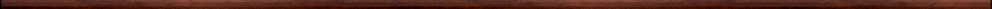Maple worksheets on finite difference methodsNumerical methods topics:

They are all compatible with Classic Worksheet Maple 10.Numerical differentiation - numdiff.mws

• Simple formulas for computing numerical approximations for 1st and 2nd derivatives of a tabulated function.
• Improving the accuracy of numerical differentiation by extrapolation.
• Constructing extrapolation formulas.

Two point boundary value problems - FDintro.mws

• The finite difference method for constructing a discrete numerical solution for a two point boundary value problem.
• An example using standard method to solve the tridiagonal system which arises when applying the finite difference method to solve a two point boundary value problem.
• Using the procedure TridiagSolve to solve the tridiagonal system arising from a two point boundary value problem.

Discrete and continuous numerical solutions for boundary value problems - desolveFD.mws

• A procedure for constructing both discrete and continuous solutions to boundary value problems: desolveFD.
The continuous solutions are constructed by means of local Taylor series approximations.

Boundary value examples involving special functions - FDspec.mws

• Examples of boundary value problems where the analytical solution involves special functions such as a Bessel functions, Legendre polynomials, etc.

Procedures for differential equations - DEsol.zip

Top of page

Main index